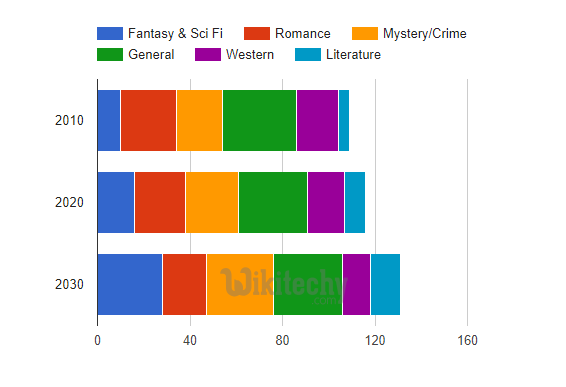## What is Stacked bar charts?

• A stacked bar chart is a bar chart that places related values atop one another.
• If there are any negative values, they are stacked in reverse order below the chart's axis baseline.
• Stacked bar charts are typically used when a category naturally divides into components.
• For instance, consider some hypothetical book sales, divided by genre and compared across time:• Stacked bar charts also support 100% stacking, where the stacks of elements at each domain-value are rescaled such that they add up to 100%.
• The options for this are isStacked: 'percent', which formats each value as a percentage of 100%, and isStacked: 'relative', which formats each value as a fraction of 1.
• There is also an isStacked: 'absolute' option, which is functionally equivalent to isStacked: true.

## Configurations

• We've used isStacked configuration to show stacked chart.